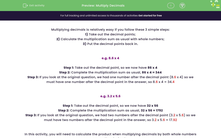# Multiply Decimals

In this worksheet, students will calculate the product when multiplying decimals by both whole numbers or another decimal. Students will practise taking out the decimal point and then re-inserting it after they have calculated the multiplication sum.Key stage:  KS 4

Year:  GCSE

GCSE Subjects:   Maths

GCSE Boards:   Pearson Edexcel, OCR, Eduqas, AQA,

Curriculum topic:   Number, Fractions, Decimals and Percentages

Curriculum subtopic:   Structure and Calculation Decimal Fractions

Popular topics:   Decimals worksheets

Difficulty level:#### Worksheet Overview

Multiplying decimals is relatively easy if you follow these 3 simple steps:

1) Take out the decimal points;

2) Calculate the multiplication sum as usual with whole numbers;

3) Put the decimal points back in.

e.g. 8.6 x 4

Step 1: Take out the decimal point, so we now have 86 x 4

Step 2: Complete the multiplication sum as usual, 86 x 4 = 344

Step 3: If you look at the original question, we had one number after the decimal point (8.6 x 4) so we must have one number after the decimal point in the answer, so 8.6 x 4 = 34.4

e.g. 3.2 x 5.6

Step 1: Take out the decimal point, so we now have 32 x 56

Step 2: Complete the multiplication sum as usual, 32 x 56 = 1792

Step 3: If you look at the original question, we had two numbers after the decimal point (3.2 x 5.6) so we must have two numbers after the decimal point in the answer, so 3.2 x 5.6 = 17.92

In this activity, you will need to calculate the product when multiplying decimals by both whole numbers or another decimal.

You should follow the three steps described above, taking out the decimal point and then re-inserting it after you have calculated the multiplication sum as usual.

### What is EdPlace?

We're your National Curriculum aligned online education content provider helping each child succeed in English, maths and science from year 1 to GCSE. With an EdPlace account you’ll be able to track and measure progress, helping each child achieve their best. We build confidence and attainment by personalising each child’s learning at a level that suits them.

Get started